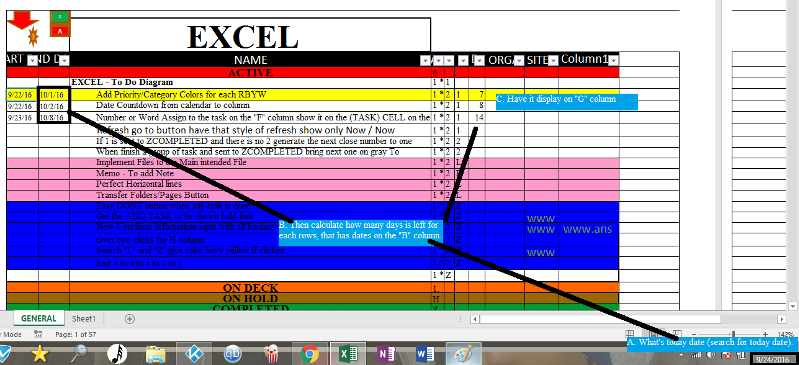Date generator for it to show Days Left for due date.

Hello guys, i have an alternative to make this possible by adding this code on the cell =IF(B6<>"",B6-TODAY(),"")

***But what i truly want is have a way where a code is created, so that i may attach it in the main page and it will calculate automatic date left from searching column "B" and display it in column "I".

again thanks for your time guys and help.Who is Participating?
I wear a lot of hats...

"The solutions and answers provided on Experts Exchange have been extremely helpful to me over the last few years. I wear a lot of hats - Developer, Database Administrator, Help Desk, etc., so I know a lot of things but not a lot about one thing. Experts Exchange gives me answers from people who do know a lot about one thing, in a easy to use platform." -Todd S.

Commented:
If you place that formula into a cell and double-click the lower right corner of the cell, it will autofill down.  That should give you what you want
Author Commented:
Hello there Aikimark, thanks for your response, i know but i am looking for another way of doing it. I have a code that i have pasted on the main page and i would like something similar like this.

``````Private Sub Worksheet_Change(ByVal Target As Range)
Dim eval As Long
If Target.Column = 2 Then
eval = Cells(Target.Row, 2).Value - Date
If eval > 0 Then
If eval = 1 Then
Cells(Target.Row, 10).Value = "Q"
Cells(Target.Row, 10).Font.Color = -16776961
ElseIf eval = 2 Then
Cells(Target.Row, 10).Value = "QQ"
Cells(Target.Row, 10).Font.Color = 26367
ElseIf eval = 3 Then
Cells(Target.Row, 10).Value = "QQQ"
Cells(Target.Row, 10).Font.Color = 26367
ElseIf eval = 4 Then
Cells(Target.Row, 10).Value = "QQQQ"
Cells(Target.Row, 10).Font.Color = 26367
ElseIf eval > 4 Then
Cells(Target.Row, 10).Value = "QQQQQ"
Cells(Target.Row, 10).Font.Color = -65536
End If
Cells(Target.Row, 10).Font.name = "Snap ITC"
Cells(Target.Row, 10).Font.FontStyle = "Bold"
Cells(Target.Row, 10).Font.Size = 8
Else
Cells(Target.Row, 10).Value = ""
End If
If Cells(Target.Row, 2) = Empty Then
Cells(Target.Row, 10).Value = ""
End If
End If

End Sub
``````

a code which will do it automatically pasted on the main page, without having it on all the cell
Commented:
This would be better
``````Private Sub Worksheet_Change(ByVal Target As Range)
Dim eval As Long
If Target.Column = 2 Then
If Cells(Target.Row, 2) = Empty Then
Cells(Target.Row, 10).Value = ""
Exit Sub
End If
eval = Cells(Target.Row, 2).Value - Date
If eval > 0 Then
If eval = 1 Then
Cells(Target.Row, 10).Value = "Q"
Cells(Target.Row, 10).Font.Color = -16776961
ElseIf eval = 2 Then
Cells(Target.Row, 10).Value = "QQ"
Cells(Target.Row, 10).Font.Color = 26367
ElseIf eval = 3 Then
Cells(Target.Row, 10).Value = "QQQ"
Cells(Target.Row, 10).Font.Color = 26367
ElseIf eval = 4 Then
Cells(Target.Row, 10).Value = "QQQQ"
Cells(Target.Row, 10).Font.Color = 26367
ElseIf eval > 4 Then
Cells(Target.Row, 10).Value = "QQQQQ"
Cells(Target.Row, 10).Font.Color = -65536
End If
Cells(Target.Row, 10).Font.name = "Snap ITC"
Cells(Target.Row, 10).Font.FontStyle = "Bold"
Cells(Target.Row, 10).Font.Size = 8
Else
Cells(Target.Row, 10).Value = ""
End If
End If

End Sub
``````
Author Commented:
Thanks Akimark, for me to be more specific i want a code so that i may put on the main page( the same page where i have the one you have polished) where it calculate the date from "B" column and is input on the "I" column showing numbers.
***Those numbers = amount of days from now to the amount of days it will take, to get to the date it is display on column "B".
***(Example) on row, column "B" = 10/4/16 on column "I" it will = 5)
***(Example) on row, column "B" = 10/9/16 on column "I" it will = 10)
***(Example) on row, column "B" = 11/4/16 on column "I" it will =36 )

Hope this is clear friend.
Author Commented:
File is on this attachment
BK-TO-DO-LIST.xlsm
Commented:
``````Private Sub Worksheet_Change(ByVal Target As Range)
Dim eval As Long
Dim colors As Variant
colors = Array(0, -16776961, 26367, 26367, 26367, -65536)
If Target.Column = 2 Then
If Cells(Target.Row, 2).Value = Empty Then
Cells(Target.Row, 10).Value = ""
Exit Sub
End If
If IsDate(Cells(Target.Row, 2).Value) Then
eval = Cells(Target.Row, 2).Value - Date
Select Case eval
Case 0
Cells(Target.Row, 10).Value = ""
Case 1 To 4
Cells(Target.Row, 10).Value = String(eval, "Q")
Cells(Target.Row, 10).Font.Color = colors(eval)
Case Is > 4
Cells(Target.Row, 10).Value = String(5, "Q")
Cells(Target.Row, 10).Font.Color = colors(5)
End Select
With Cells(Target.Row, 10).Font
.name = "Snap ITC"
.FontStyle = "Bold"
.Size = 8
End With
Cells(Target.Row, 9).Value = eval
End If
End If

End Sub
``````

Experts Exchange Solution brought to you by

Facing a tech roadblock? Get the help and guidance you need from experienced professionals who care. Ask your question anytime, anywhere, with no hassle.

Author Commented:
thanks a mil aikimark, works like a charm... :)
Author Commented:
Hey there aikimark, quick question is it possible to have this code to work in a way, that when i click on the refresh button the code will re-check all the dates on the "B" column and re-arrange dates left on the "I" column ?
Commented:
Possibly.  What do you mean by "rearrange"?
Author Commented:
Hey aikimark i have made a video for a better visual description if you may, please check it out on the link provided.

http://www.screencast.com/users/Omarantonio1231/folders/Refresh%20Dates/media/f19fa1e9-3805-40f9-8bb7-ced979e14608
Commented: